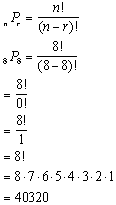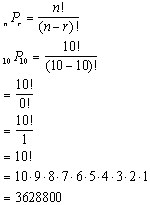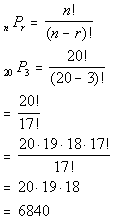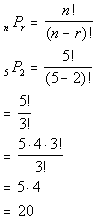TitleCollege Algebra
Tutorial 56: PermutationLearning Objectives

 After completing this tutorial, you should be able to: Use permutations to solve a counting problem involving order.Introduction

 In this tutorial we will be going over permutations.  Permutations are an off shoot of the Fundamental Counting Principle. If you need a review on the Fundamental Counting Principle, feel free to go to Tutorial 55: The Fundamental Counting Principle.  Permutations specifically count the number of ways a task can be arranged or ordered. I think you are ready to go off into the wonderful world of permutations, have fun!Tutorial

 Factorial !

 The factorial symbol is the exclamation point: ! So if I wanted to write 7 factorial it would be written as 7!. In general, n! = n(n - 1)(n - 2)(n - 3)...(1) Most, (if not all), of you will have a factorial key on your calculator.  It looks like this: ! If you have a graphing calculator, it will be hidden under the MATH menu screen and then select your Probability screen - there you should find ! Some calculators don’t have one, so I will show you how to simplify the problems in case you don’t have that key on your calculator. 0!  Has a special definition attached with it.  0! = 1Example 1: Find 7!

 If you have a ! key on your calculator you simple press 7 and then ! and in some cases you may have to also press your enter or = key. If you don’t have this key you will have to enter the definition in as follows: 7! = (7)(6)(5)(4)(3)(2)(1) = 5040 Either way 7! = 5040.

 We needed to know about factorial because it is used the formula for permutation, which is our next topic.

 Permutation An ORDER of arrangements of r objects, without repetition, selected from n distinct objects is called a permutation of n objects taken r at a time, and is denoted asIn other words, when you need to count the number of ways you can arrange items where ORDER is important, then you can use permutation to count.  For example, you may want to know how many ways to pick a 1st, 2nd, and 3rd place winner from 10 contestants.  Since you are arranging them in order, you could use a permutation to do this.  Or if you were wanting to know how many ways your committee could pick a president, a vice president, a secretary, and a treasurer, you could use permutations.Example 2:  In how many ways can 8 CD’s be arranged on a shelf?

Since we are arranging these CD’s that means order is important.  So we can use permutations to help us out here.

First we need to find n and r

 n is the number of CD's we have to choose from.   What do you think n is in this problem? If you said n is 8 you are correct!!!  There are 8 CD's in this problem.   r is the number of CD’s we are using at a time.  What do you think r is? If you said r is 8, pat yourself on the back!!  We are arranging all 8 CD's on the shelf.

Putting this into the permutation formula we get:*n = 8, r = 8       *0! = 1    *Expand out 8!

 If you have a factorial key, you can put it in as 8! divided by 0! and then press enter or =. If you don't have a factorial key, you can simplify it as shown above and then enter it in.  It is probably best to simplify it first, because in some cases the numbers can get rather large, and it would be cumbersome to multiply all those numbers one by one. Wow, this means there are 40320 different ways to arrange those 8 CD’s, that’s a lot.Example 3:  If a softball league has 10 teams, how many different end of the season rankings are possible?  (Assume no ties).

Since we are ranking these teams that means order is important.  So we can use permutations to help us out here.

First we need to find n and r :

 n is the number of teams we have to choose from.   What do you think n is in this problem? If you said n is 10 you are correct!!!  There are 10 teams in this problem.   r is the number of teams we are ranking at a time.  What do you think r is? If you said r is 10, pat yourself on the back!!  We are ranking all 10 teams.

Putting this into the permutation formula we get:*n = 10, r = 10       *0! = 1    *Expand out 10!

 If you have a factorial key, you can put it in as 10! divided by 0! and then press enter or =. If you don't have a factorial key, you can simplify it as shown above and then enter it in.  It is probably best to simplify it first, because in some cases the numbers can get rather large, and it would be cumbersome to multiply all those numbers one by one. Wow, this means there are 3,628,800 different ways to rank those 10 teams, that’s a lot.Example 4:  In how many ways can a sorority of 20 members select a president, vice president and treasury, assuming that the same person cannot hold more than one office.

Since we are choosing offices, which is a way to rank members, that means order is important.  So we can use permutations to help us out here.

First we need to find n and r :

 n is the number of members we have to choose from.   What do you think n is in this problem? If you said n is 20 you are correct!!!  There are 20 members in this problem.   r is the number of members we are selecting for offices at a time.  What do you think r is? If you said r is 3, pat yourself on the back!!  There are 3 offices.

Putting this into the permutation formula we get:*n = 20, r = 3       *Expand 20! until it gets to 17! ( which is the ! in den) *Cancel out 17!'s

 If you have a factorial key, you can put it in as 20! divided by 17! and then press enter or =. If you don't have a factorial key, you can simplify it as shown above and then enter it in.  It is probably best to simplify it first, because in some cases the numbers can get rather large, and it would be cumbersome to multiply all those numbers one by one. Wow, this means there are 6840 different ways to select the three officers, that’s a lot.Example 5:  How many different arrangements can be made using two of the letters of the word TEXAS if no letter is to be used more than once?

Since we are arranging letters, this means order is important.  So we can use permutations to help us out here.

First we need to find n and r :

 n is the number of letters we have to choose from.   What do you think n is in this problem? If you said n is 5 you are correct!!!  There are 5 letters in TEXAS.   r is the number of letters we are using at a time.  What do you think r is? If you said r is 2, pat yourself on the back!!  We are using 2 letters at a time.

Putting this into the permutation formula we get:*n = 5, r = 2       *Expand 5! until it gets to 3! ( which is the ! in den) *Cancel out 3!'s

 If you have a factorial key, you can put it in as 5! divided by 3! and then press enter or =. If you don't have a factorial key, you can simplify it as shown above and then enter it in.  It is probably best to simplify it first, because in some cases the numbers can get rather large, and it would be cumbersome to multiply all those numbers one by one. This means there are 20 different 2 letter arrangements.Practice Problems

 These are practice problems to help bring you to the next level.  It will allow you to check and see if you have an understanding of these types of problems. Math works just like anything else, if you want to get good at it, then you need to practice it.  Even the best athletes and musicians had help along the way and lots of practice, practice, practice, to get good at their sport or instrument.  In fact there is no such thing as too much practice. To get the most out of these, you should work the problem out on your own and then check your answer by clicking on the link for the answer/discussion for that  problem.  At the link you will find the answer as well as any steps that went into finding that answer.Practice Problems 1a - 1c: Solve using permutations.

 1a. A company issues a questionnaire whereby each employee must rank the 5 items with which he or she is most satisfied.  The items are wages, work environment, vacation time, job security, supervisors, health insurance, break time, and retirement plan. The ranking is to be indicated by the numbers 1, 2, 3, 4 and 5, where 1 indicates the item involving the greatest satisfaction and 5 the least.  In how many ways can an employee answer this questionnaire? (answer/discussion to 1a)

 1b. A key pad lock has 10 different digits, and a sequence of 5 different digits must be selected for the lock to open.  How many key pad combinations are possible? (answer/discussion to 1b)

 1c.  In how many ways can 7 books be arranged on a shelf? (answer/discussion to 1c)Need Extra Help on these Topics?

 Go to Get Help Outside the Classroom found in Tutorial 1: How to Succeed in a Math Class for some more suggestions.

Last revised on May 20, 2011 by Kim Seward.